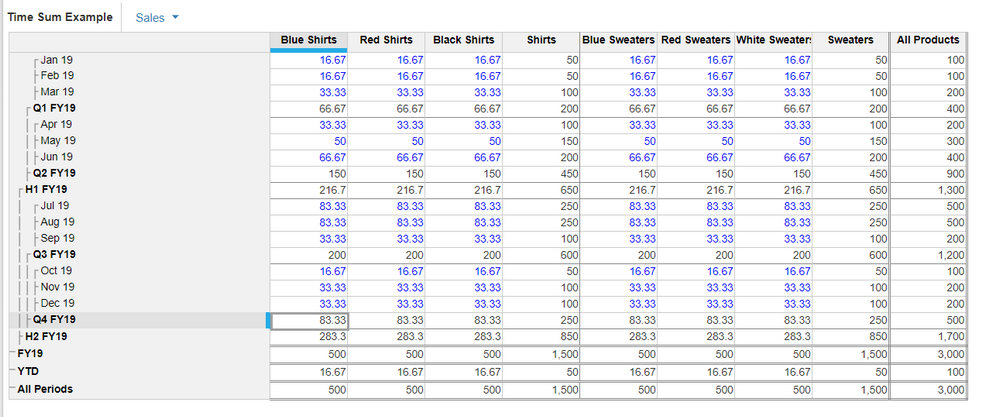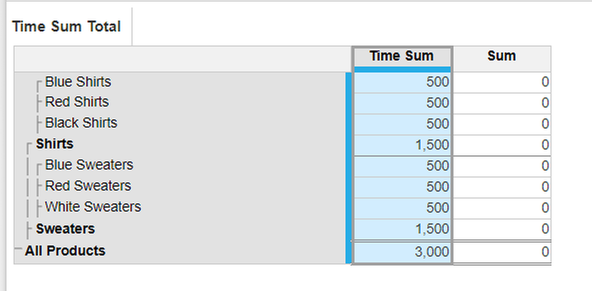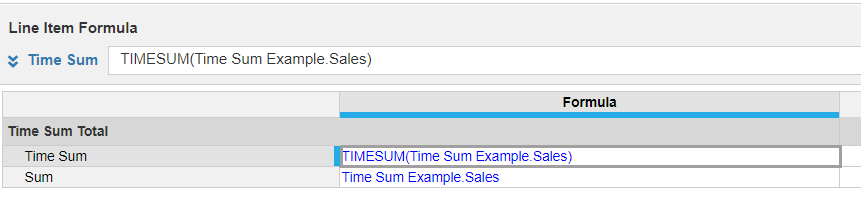# Sum values based on periodHi,

Need a help to build a formula, have attached a screenshot for reference. Formula Needs to be build in Revenue Seasonality which need to take the values from Revenue Column and doing the sum of Periods.

For Example: Period 1 amounts: 112+ 95. Needs to be clubbed as 207 and shown in Revenue Seasonality against all the Period 1. Similar goes for rest of the periods.

Thanks.

Tagged:

•Several ways you can accomplish this.

1. First is to use TIMESUM.
2. Second is to use a boolean on each of the time periods you want included. Can be dynamic if you want Then multiply the value times the 1 or 0 to sum up the preferred sales.

Here's an example of TIMESUM:

Sales by product:Sales using TIMESUM. Note that the "Sum" line item doesn't work because you can't directly refer to the line item on the Time dimension.Blueprint mode•Since your period is a list formatted line item , it establishes a mapping between time and period list,

so simply use Revenue[sum:souce.period] and it will aggregate against period dimension in target module